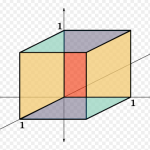# C# Linear Programming

In NMath two classes are used to describe a linear programming (LP) problem. The first class LinearProgrammingProblem encapsulates a standard LP problem and the second, MixedIntegerLinearProgrammingProblem, encapsulates a LP problem which may contain integer or binary constraints.

Note that with the release of NMath 7, all analysis types were unified into the CenterSpace.NMath.Core namespace and the CenterSpace.NMath.Analysis namespace was deprecated.Class PrimalSimplexSolver solves linear programming problems using the primal simplex method. DualSimplexSolver uses the dual simplex method. The simplex method solves LP problems by constructing an initial solution at a vertex of a simplex, then walking along edges of the simplex to vertices with successively higher values of the objective function until the optimum is reached.

### Linear Programming Documentation

For more documentation on the NMath linear programming API, see:

### Linear Programming Code Examples

All NMath libraries include extensive code examples in both C# and Visual Basic. Studying these examples is one of the best ways to learn how to use NMath libraries. For more information on linear programming, see:

• LinearProgrammingExample [C#]  [VB]
Example showing how to solve a linear programming problem.
• PrimalDualSimplexExample [C#]  [VB]
Example showing how to solve the Klee Minty cube linear programming problem using both primal and dual simplex methods.
• MixedIntLinearProgrammingExample [C#]  [VB]
Example showing how to solve a linear programming problem where one of the variables is constrained to have an integer value.
• BinaryLinearProgrammingExample [C#]  [VB]
Example showing how to solve a LP problem where all the variables are binary.

### Try a Free EvaluationIf you are interested in evaluating the  simplex solver class in NMath, we offer a free trial version, for a 30-day evaluation period. This trial version is a fully featured distribution of NMath with no limitations. In only a few minutes you can be enjoying the power of NMath.Orders may be placed through our secure online store using either google checkout or paypal checkout. Our sales staff would be happy to help you with any questions that you may have about our products.  We are looking forward to working with you!